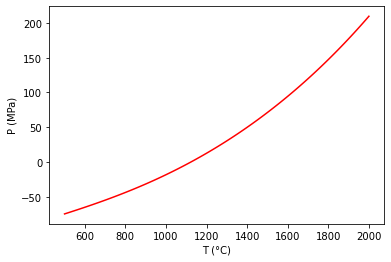# Plot a Reaction between Stoichiometric Phases¶

```from thermoengine import phases
from thermoengine import model
import numpy as np
import matplotlib.pyplot as plt
%matplotlib inline
```

## Get access to a thermodynamic database (by default, the Berman (1988) database).¶

```modelDB = model.Database()
```

## To print a list of all of the phases in the database, execute:¶

```print(thermoDB.all_purephases_df.to_string())
```

## Specify a new reaction by indicating reactants, products.¶

forsterite + quartz = 2 enstatite

```reaction = modelDB.get_rxn(['Fo','Qz','cEn'],[0,0,0],[-1,-1,2])
```
`<string>:6: DeprecationWarning: Calling nonzero on 0d arrays is deprecated, as it behaves surprisingly. Use atleast_1d(cond).nonzero() if the old behavior was intended. If the context of this warning is of the form arr[nonzero(cond)], just use arr[cond].`

## Set up temperature bounds, and calculate the univariant curve.¶

```Tlims = 273.15+np.array([500,2000])
univariant_line = reaction.trace_boundary(Tlims=Tlims,Nsamp=100)
```

## Now, plot up the results.¶

```plt.plot (univariant_line-273.15, univariant_line/100.0, 'r-')
plt.ylabel('P (MPa)')
plt.xlabel('T (°C)')
plt.show()
```